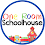## Thursday, February 4, 2016

### Comparing Fractions Using the Butterfly Method

Do your kids struggle with comparing fractions with unlike denominators?  Most do because it's so hard.  Since I started teaching my kids using the butterfly method, they are all pros.

I start the lesson by getting the kids to think about the denominator and what it really means.  I teach them the bigger the denominator, the small the piece that each person gets.  Once my kids understand this concept, they can easily compare fractions with like denominators.   The problem comes when the denominators are different.

I have my kids draw a butterfly shape with the fractions.  This just helps them keep their numbers straight when they cross multiply.

### How does cross multiplying work?

We are finding equivalent fractions with like denominators.  When the denominators are the same, you compare the numerators.  Easy, right?Share:

1.I tell 4th & 5th grade teachers to not teach the butterfly method because students are just learning a "trick" rather than learning why this method truly works. I have had 6th and 5th grade teachers tell me that students try to cross multiply when they are to just multiply fractions because they are confused. So, they begin to apply these in places where they do not work. And they do not promote understanding of fractions." It is better to teach students to compare fractions to benchmark fractions or find a common denominator.

2.If you are teaching it as a trick, then I totally agree. When I teach it, I explain to the kids that we are finding equivalent fractions with a common denominator. When fractions have a common denominator, then you are able to compare using the numerator.

3.It is not a trick if you show them how solving the same problem by finding a least common denominator will lead to two new numerators which match the cross-products from the butterfly method.

1.I agree with all of you. Teach benchmark fractions and common denominators too but this gives students another strategy to use. Would we call the standard algorithms for operations, tricks? No, because we teach them why it works.